# Fredholm eigenvalue of a Jordan curve

(diff) ← Older revision | Latest revision (diff) | Newer revision → (diff)

Letbe a smooth Jordan curve (of class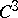) in the complex-plane,its interior,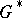its exterior. Then, let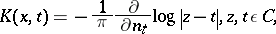be the corresponding classical Neumann kernel with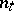the interior normal. The Fredholm eigenvalueofis the smallest eigenvalue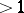of this kernel.

This eigenvalue plays an important role for the speed of the successive approximation solution of several problems, such as the integral equation with this kernel corresponding to the Dirichlet problem and several integral equations to construct the conformal Riemann mapping function of[a1].

For arbitrary Jordan curves, there is the following characterization of the Fredholm eigenvalue[a4]: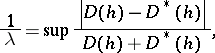where the supremum is over all functionsthat are continuous in the extended plane and harmonic inand in, with corresponding Dirichlet integrals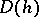and(cf. also Dirichlet integral).

One has: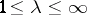, with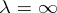if and only ifis a circle, and with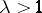if and only ifis a quasi-circle [a4], [a2]. In some sense,is a measure for the deviation offrom a circle.is invariant under Möbius transformations (cf. also Fractional-linear mapping).

The exact value ofis known for several special Jordan curves: e.g. ellipses, some Cassinians, triangles, regular-gons, and rectangles close to a square.

There is also the following characterization of, using the Riemann mapping of. Without loss of generality one may assume thatis the image of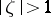under a univalent conformal mapping (cf. also Conformal mapping)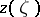of the form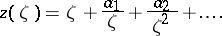One can then calculate the so-called Grunsky coefficients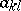in the development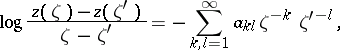Then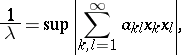where the supremum is taken over all complex numbers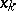with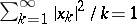. This gives also a procedure to evaluatenumerically [a2].

Hence one obtains simple upper estimates for, of course. There are many other such estimates in which the mappingis involved [a2]. As a simple consequence, there is the following very useful inequality for Jordan curveswith corners [a2]: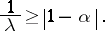Here,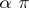denotes the angle at the corner.

For largeone finds [a2] thatmust be contained in an annulus with radiiand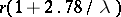such thatseparates the boundary circles with these radii. However, in the other direction,can be close toeven thoughlies, in the same manner, in an annulus for which the quotient of the radii is arbitrarily close to.

There are also several lower estimates from M. Schiffer and others for[a1], [a2], [a4]. If, for example,is the image of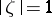under a univalent conformal mapping of an annulus(), thenL.V. Ahlfors noted a remarkable interaction between the theory of Fredholm eigenvalues and the theory of quasi-conformal mapping: If there is a-quasi-conformal reflection at(i.e., a sense-reversing-quasi-conformal mapping of the extended plane which leavespointwise fixed), then [a2], [a4]The question of equality gives rise to interesting connections with the theory of extremal quasi-conformal mappings (connected with the names of O. Teichmüller, K. Strebel, E. Reich; cf. [a2]).

From this Ahlfors inequality one obtains almost immediately [a2], [a4]: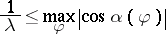ifis smooth and starlike with respect to the interior point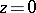, wheredenotes the angle between the ray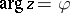and the tangent at the point ofwith.

For a theory of Fredholm eigenvalues for multiply-connected domains, see [a3].

How to Cite This Entry:
Fredholm eigenvalue of a Jordan curve. Encyclopedia of Mathematics. URL: http://encyclopediaofmath.org/index.php?title=Fredholm_eigenvalue_of_a_Jordan_curve&oldid=17984
This article was adapted from an original article by Reiner KÃ¼hnau (originator), which appeared in Encyclopedia of Mathematics - ISBN 1402006098. See original article